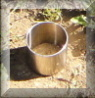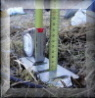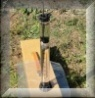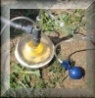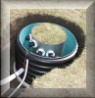# Unsaturated hydraulic conductivity: Mini Disk Infiltrometer

The Mini Disk Infiltrometer (Decagon Devices, Inc.) is a very handy, manually operated device to measure unsaturated hydraulic conductivity at tensions between -0.5 cm and -6 cm. It consists of two chambers, which are filled with water (see Fig 1).
 The upper chamber (bubbling chamber) controls the suctions. Water for infiltration is stored in the lower chamber. There is a porous, sintered, stainless steel disk at the bottom of the lower chamber. The disk is 0.3 cm thick and has a diameter of 4.5 cm. In the field a disk with such a small parameter is relatively easy to set up on the soil surface, which needs to be smooth and levelled.Fig 1. Mini Disk Infiltrometer (Decagon Devices, Inc., 2005)
However, only a small part of the soil is measured, which does not fulfil the requirements of the representative volume.
The amount of water, which can be infiltrated is 135 cm3. The time interval for manual readings needs to be determined according to the soil type being tested, and the tension. The recommended interval in the User’s manual for sand is 2 to 5 s, for silt loam 30 s and for tight clay 30 to 60 minutes.

Watch the video in order to see the infiltration taking place.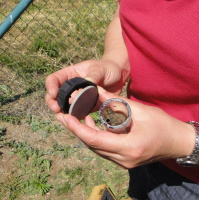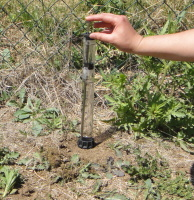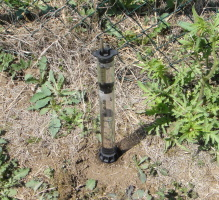## Measurement procedure

A special care needs to be taken to the preparation of the soil surface. Good hydraulic contact between the infiltrometer disk and the infiltration surface is essential for correct measurement. If the surface is not smooth, a thin layer of silica sand can be used as a contact material. When filling the infiltrometer with water, start with the bubbling chamber and then fill also the water reservoir tube. Make sure that water is not leaking from anyplace around the disk. Set the suction rate (depending on the soil). According to the User’s manual, a suction rate of 2 cm should be adequate for most soils. To start the experiment, record the initial water level in the reservoir tube, place the infiltrometer on the surface, and start the stopwatch. The water level needs to be recorded at regular time intervals (see the data record form for the Mini Disk Infiltrometer on the right).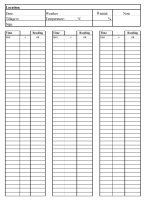## Data analysis

To determine the unsaturated hydraulic conductivity for the applied tension, a method proposed by Zhang (1997) can be applied. Cumulative infiltration vs. time is measured and then fitted with the following function (Philip, 1957):where C1 (L T-1) and C2 (L T-1/2) are parameters related to hydraulic conductivity and soil sorptivity, respectively, I (L) is the cumulative infiltration, and t (T) the time.

The hydraulic conductivity of the soil K at the particular applied tension h is then calculated as follows:where C1 is the slope of the curve of the cumulative infiltration vs. the square root of time, and A is a value relating the van Genuchten parameters (van Genuchten, 1980) for 12 soil texture classes to the radius of the disk and applied tension. The A parameters can be obtained from Tab 1 or calculated according to the following formulae using the van Genuchten parameters also listed in Tab 1.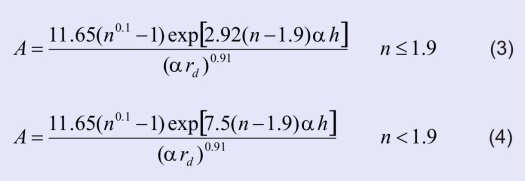where n (-) and α (L-1) are the van Genuchten parameters, rd (L) is the disk radius and h (L) is the applied tension.

Tab 1. Van Genuchten parameters and values of A for the Minidisk Infiltrometer (Decagon Devices, Inc., 2005)The parameter C1 is determined in the following way: Plot the graph of cumulative infiltration I in cm (recalculated from the volume infiltrated by using the infiltration area) and square root of time t; the points need to be fitted by a polynomial function of the second degree: quadratic equation y = ax2+b, where a is parameter C1, see Fig 2. Use the Excel option "Add trendline" and make options to show the regression equation with y = 0 and to show the coefficient of determination.
The unsaturated hydraulic conductivity is then calculated based on equation (2).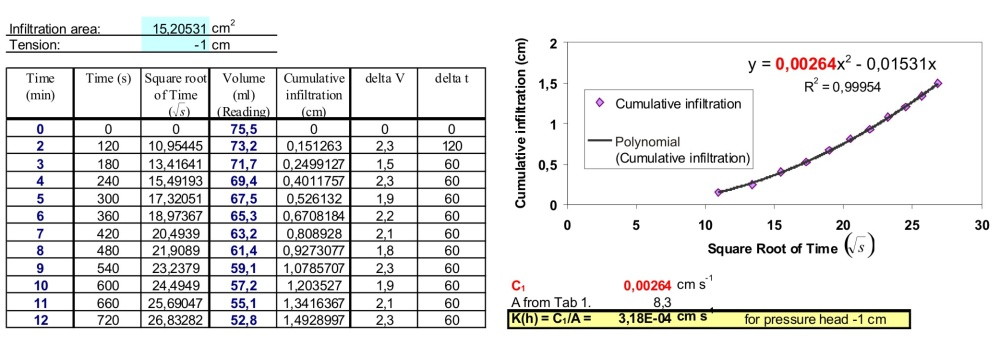Fig 2. Example of data analysis. Similar sheet of solution can be downloaded from http://www.decagon.com.

More extensive discussion of tension infiltrometer measurement and analysis is given in Dane and Topp (2002).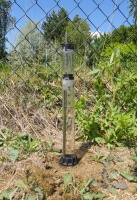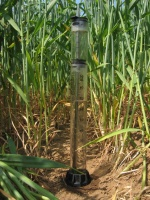### References

Dane, J.H., and G.C. Topp (eds.) 2002. Methods of Soil Analysis. Part 4 — Physical Methods, Soil Science Society of America Book Series, Vol. 5, p. 888-896.

Decagon Devices, Inc., (2005). Minidisk infiltrometer, User’s manual.

Philip, J.R. (1957) The theory of infiltration: 4. Sorptivity and algebraic infiltration equations Soil Science 84, 257-264.

van Genuchten, M.T. 1980. A Closed-form Equation for Predicting the Hydraulic Conductivity of Unsaturated Soils. Soil Science Society of America Journal. 44(5). 892-898.

Zhang, R. (1997) Determination of soil sorptivity and hydraulic conductivity from the disk infiltrometer. Soil Science Society of America Journal, 61, 1024-1030.

Note: Fig 1 and Tab 1 are taken from the above mentioned guide "Mini Disk Infiltrometer. User's manual."